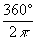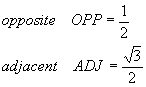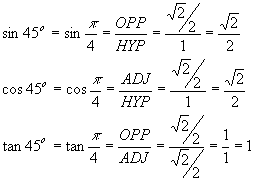Trigonometric Functions of Common Angles

The fundamental ideas needed to understand sines and cosines can be put into one graph, the unit circle:Here we have a circle of radius r = 1 (hence "unit circle"), a point (x, y) on that circle, and perpendiculars from the point to the x and y axes. The line from the origin (0, 0) to the point (x, y) is considered a vector and is usually just labeled with the endpoint (x, y). This vector forms an angle q (Greek theta) with the x-axis. The line segment from (0, 0) to (x, y) is the longest of the three sides of the triangle and is called the hypotenuse. Notice that the point on the x-axis where the perpendicular meets the axis is marked "x = cos q" and the corresponding point on the y-axis is marked "y = sin q". These two points represent the meaning of the sine and cosine of the angle, normally understood as the adjacent and opposite respectively.

Angles are given either in degrees (1 complete circle = 360o) or radians (1 complete circle = 2p). For pure mathematics, radians are preferred, although both measures of angles are in very common use. Generally speaking, when we are interested in sine and cosine as functions (of the angle q), we use radians. Radians are pure numbers, the same as 0, 1, 4.3874653 and p = 3.14159…, while degrees carry units (denoted with the degree sign o). Degrees require special handling – if you use a calculator to calculate the value of trig functions of an angle, you have to make sure you tell your calculator which units, degrees or radians, you are using before you do the calculation.

It is reasonably easy to convert between degrees and radians. We gave the number of each unit in one complete circle above, so:Thus, to convert degrees to radians, multiply byTo convert radians to degrees, multiply byExample

Solution

Using the first of the two conversion factors above,Two details should be pointed out here.

The first is that we did not write "radians" after the answer – the lack of units is typical of pure numbers and is deliberate. If we know the number is an angle and there is no degree sign after it, the likelihood is that the angle has been expressed in radians. The presence of p in the expression is another clue that the angle is expressed in radians. This is an irrational number, and expressing it exactly requires that it be in terms of a multiple of p.

The second detail is how we did the calculation – notice that the degree sign appears once in the numerator (we deliberately expressed 14 as the improper fraction 14/1 to give it an explicit denominator) and once in the denominator. The degrees cancel out – a valuable memory aid for those of us who can’t remember which conversion factor to use!

Example

Convert 3p/4 to degrees.

Solution

Using the second of the two conversion factors above,Notice that angles in radians are most often expressed as multiples of p, which helps us decide which conversion factor to use. The important point is that we wanted our answer to be in degrees, which meant that the degree unit needed to be in the numerator. It is possible for us to talk about angles in radians which are not expressed as multiples of p, but in practice such angles occur infrequently.

The coordinate system (also known as the x-y plane) is divided into four regions, or quadrants:

 Quadrant Allowed angles q (min, max) in degrees; radians Allowed values of x = cos q Allowed values of y = sin q I (0, 90); (0, p/2) x ³ 0 y ³ 0 II (90, 180); (p/2, p) x £ 0 y ³ 0 III (180, 270); (p, 3p/2) x £ 0 y £ 0 IV (270, 360); (3p/2, 2p) x ³ 0 y £ 0

It is handy to take note of the boundaries between the quadrants:

(x, y) = (1, 0): Angle with the x-axis: q = 0 Þ cos q = cos 0 = 1; sin q = sin 0 = 0.

(x, y) = (0, 1): Angle with the x-axis: q = 90o = p/2 Þ cos q = cos p/2 = 0; sin q = sin p/2 = 1.

(x, y) = (-1, 0): Angle with the x-axis: q = 180o = p Þ cos q = cos p = -1; sin q = sin p = 0.

(x, y) = (0, -1): Angle with the x-axis: q = 270o = 3p/2 Þ cos q = cos 3p/2 = 0; sin q = sin 3p/2 = -1.

These turn out to show the extreme values of both sine and cosine functions. It is imperative to commit them to memory, along with several additional common angles. These common angles are most often described in the first quadrant only (acute angles); common angles in the rest of the quadrants are obtained from the acute angles by various elementary operations that we will describe in a separate article.

Acute angles have measure less than 90o = p/2. There are two important right triangles (where one of the internal angles is exactly 90o = p/2 and the other two are acute) used as examples to study the trig functions of the acute angles within them. The first is the 30o/60o triangle, the second is the 45o triangle.In keeping with the unit circle concept, we will set the hypotenuse to have unit length, HYP = 1. The orientation of the triangle suggests that the angle we are currently studying is the q = 30o = p/6 angle at the lower left corner of the triangle. If this triangle was placed on the above graph with this corner at the origin, the base of the triangle would lie along the x-axis and the top right corner would lie on the unit circle. As it turns out, the remaining sides of the triangle areWe are interested in the trigonometric functions of this 30o angle. By definition,Now, if we take this triangle and re-orient it so that the 60o angle is at the bottom left corner,we can do a similar exercise and find the trig functions for this angle as well. Here, HYP = 1 again, but the other sides of the triangle have been reversed, so thatThe angle is q = 60o = p/3. Thus, the common trig functions are:back to common angles

The last of the common triangles is the 45o = p/4 triangle, where both of the acute angles are the same:Again, HYP = 1, and the remaining sides areThe common trig functions areback to common angles

We mentioned above the definition of a right triangle - it contains a right (90o) angle with the other two acute. There are several other facts about triangles that may be helpful in later discussions.

1. The sum of the three angles in a triangle is always 180o. Thus, if the triangle is a right triangle, the other two angles must sum to exactly 90o. Such angles are called complementary angles.
2. If two triangles have the same three angles but different lengths of corresponding sides (i.e., the triangles look the same, but one is bigger than the other), then the triangles are said to be similar. We are interested (for the most part) in right triangles in trigonometry and in particular those right triangles whose hypotenuses have length one (1). Studying these triangles with unit hypotenuse allows us to fit them into the unit circle. Since similar triangles have the same angles, the ratios that are used to calculate trig functions are always the same, and so we can standardize and simplify our studies considerably. In other words, studying the unit hypotenuse triangles gives the same trig information as all similar triangles. The only difference is the length of the sides, and the same factor can be used to multiply the lengths of the sides to go from a unit hypotenuse triangle to any similar triangle.
3. Every triangle can be broken into no more than two right triangles by drawing an appropriate perpendicular from one vertex to the opposite side. This fact can be used to analyze non-right triangles using the methods of trigonometry. More on this subject later.